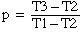Subject: Solve For Two Variables How do I solve for %1 and %2 in the following formula when T1, T2 and T3 are known? %1 and %2 are ratios of the same element, therefore %1 + %2 = 100% (%1 x T1) + (%2 x T2) = T3 The formula calculates the resulting temperature from the percentage ratios of two streams with individual temperatures. The three temperatures are known, calculating the two percentages directly from the temperatures is the mission. Hi, You have said that "%1 and %2 are ratios of the same element", so I would write them as ratios, say p and q, where p + q = 1. Thus q = 1 - p. Hence (p x T1) + (q x T2) = T3 p x T1 + (1 - p) x T2 = T3 Expanding and collecting terms containing p gives p x T1 + T2 - p x T2 = T3 p x (T1 - T2) = T3 - T2 Thusand q = 1 - p While this expression looks simple and easy to use it might give spurious results. If T1 and T2 are close to the same value then T3 will also have a value close to T1 and T2. In this case T1 - T2 and T3 - T2 will be close to zero and may be in the range of your measurement error. In this case the calculated p will be meaningless. Cheers, Penny Go to Math Central